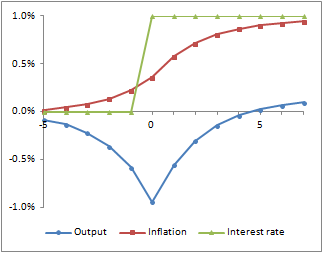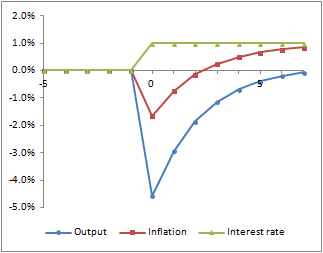Sunday, 15 November 2015

Neo-Fisherism and Term Debt

I just got round to having a look at John Cochrane's recent paper on the neo-Fisherian hypothesis, that higher nominal interest rates raise inflation.  A main theme of this paper is the issue of whether there is a theoretical basis for thinking that, even if this holds as a long-run equilibrium condition, the short run impact is the reverse.  It is commonly believed that the initial response to a higher interest rate will be lower inflation; Cochrane looks at whether we are right to think this.

The paper makes a good case that the basic New Keynesian model does not in fact imply the conventional result.  Cochrane also looks at several possible variations but concludes that these do not providing a convincing case for an initial deflation.

In my view, there is a very simple reason explanation of why we might expect an initial deflation and it's to do with term debt.  This is easy to see within Cochrane's model.

He starts with two of the three equations from the basic New Keynesian model: an equation for output (y) based on the nominal interest rate (i) and the inflation rate (π) and a NK Phillips curve.

yt = E[yt+1] { β ( 1 + it ) / ( 1 + E[πt+1] ) }

1 + πt = ( 1 + E[πt+1] )β ytκ

The third equation (the central bank reaction function) is replaced with an interest rate peg.

Cochrane also considers the inclusion of a public sector budget constraint:

Bt = ( Bt-1 -  st pt ) ( 1 + it )

where B is the nominal stock of single period government debt by face value (which we'll call bills), s is the real primary public sector surplus and p is the price level.  This accounting identity can be extrapolated to show that the current real value of public debt is equal to the discounted value of future primary surpluses.  In turn, taking the historical level of public debt together with expected future surpluses and real interest rates gives us a current equilibrium price level, as follows:

pt = Bt-1 / E[St]

where E[S] is the expected present value of future surpluses.

Cochrane uses this to distinguish between the multiple equilibria that are admitted by the simple two equation model, but finds that this does not convincingly point to an initial deflation.

From looking at how SFC models work, the thing that I would do at this point is try to incorporate some term debt.  The easiest way to do that here is to suppose that some part of the public debt consists of an annuity which pays a fixed nominal amount.  The public sector budget constraint (assuming no new issue of annuities) is now:

Bt = ( Bt-1 + a -  st pt ) ( 1 + it )

where a is the nominal value of the annuity payment.  The equilibrium price level now becomes:

pt = ( Bt-1 + At  + a ) / E[St]

where A is the nominal present value of the future annuity stream based on expected future interest rates (and assuming here that this is valued and discounted at the same rate as is paid on bills).

Examining this formula, we can see why a rise in expected nominal interest rates might create an initial deflationary effect.  Whilst the interest rate change has no impact on the value of bills, it does effect the value of the annuity.  A rise in expected nominal interest rates reduces the value of the annuity and therefore reduces the equilibrium price level.  If annuities make up a sufficient part of the public debt, this results in an initial deflation.

The charts below show what this looks like in Cochrane's model.  I have used the same parameters and the charts are the equivalent of Cochrane's Figure 1, but with 25% by value of public debt assumed to be in the form of annuities.

The first chart shows the result of an expected increase in the nominal rate.  This chart is essentially the same as Cochrane's Figure 1.  This is what we would expect, because if everything pans out in line with expectations, there is no difference between bills and annuities.The second chart shows the impact of an unexpected rate increase.  This is very different.  Not only do we get a much greater fall in real output but we now also get a temporary fall in inflation.  This is the result of the shock revaluation of term debt.Of course, this result should be of no surprise to anyone familiar with SFC modelling.  These dynamics are typical of what happens in models which combine long run and short run debt and are relatively insensitive to assumptions about expectation formation or inter-temporal preference.

[Edited to conform a bit more closely to Cochrane.]

1.Is this the model that David Andolfatto thought was "very very interesting?" Did you email him your code?

O/T: Nick, I saw your comment on Nick Rowe's "framework" post. Do you also have a framework you work from? How would you describe it? Here's a criticism of Rowe's framework I read in another blog, and I'm curious what your opinion is:

"Nick Rowe jumps in with something that is also not a conceptual framework, but rather a vague model or a collection of priors. Among other things his framework for understanding macroeconomics -- where one of the big unsolved problems is what is a recession -- includes a specific definition and mechanism of a recession."

"If I said I was a doctor studying Alzheimer's and my conceptual framework included a tenet that Alzheimer's disease was defined by amyloid plaque build-up (rather than, say, the stereotypical symptom of memory loss) and lo and behold I put up some micrographs of amyloid plaque build-up in a neuron and said that caused Alzheimer's ... exactly what is my conceptual framework helping me understand?"

"A macroeconomic framework should not postulate what a recession is. You should use the framework to figure out what a recession is."

1.Hi Tom,

No - David was interested in my take on the Diamond growth model, in my post from February. We've exchanged some brief emails, but he hasn't had a chance to look at it in any detail.

I think Nick Rowe's framework is fine for thinking about issues to do with the importance of monetary exchange. It would not be adequate for considering other issues. In my opinion, it's a mistake to limit yourself to one framework (and I'm not suggesting that Nick does so). You need to choose frameworks suitable to the task and ideally to look at things from multiple perspectives.

2.Thanks Nick. So I take it you use a variety of frameworks, perhaps including the one that Nick Rowe outlined?

3.Yes, I'd have no problem using Nick's framework as one of a number of different approaches.